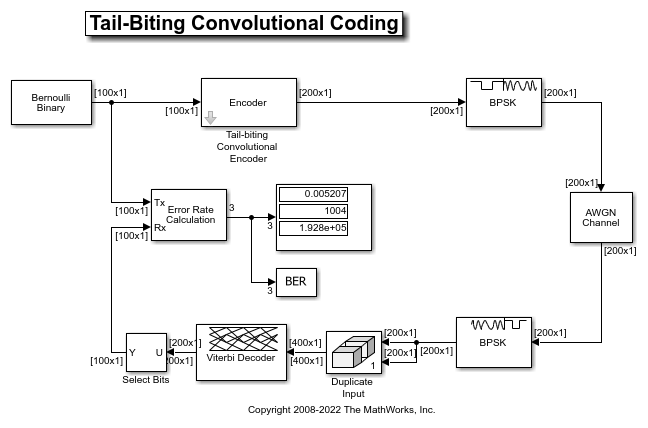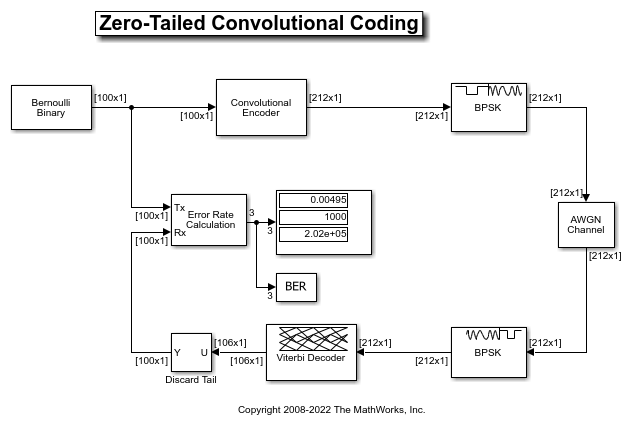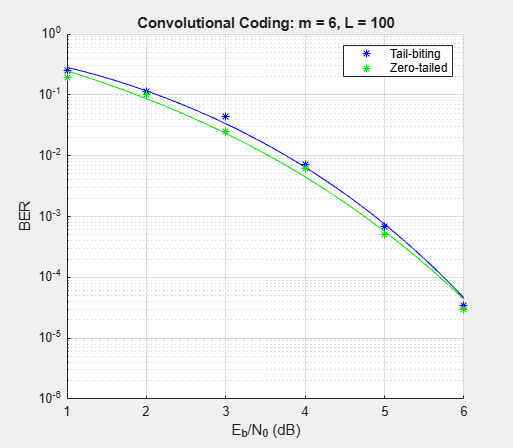# Tail-Biting Convolutional Coding

This model shows how to use the `Convolutional Encoder` and `Viterbi Decoder` blocks to simulate a tail-biting convolutional code. Terminating the trellis of a convolutional code is a key parameter in the code's performance for packet-based communications. Tail-biting convolutional coding is a technique of trellis termination which avoids the rate loss incurred by zero-tail termination at the expense of a more complex decoder [ 1 ].

The example uses an ad-hoc suboptimal decoding method for tail-biting decoding and shows how the encoding is achieved for a feed-forward encoder. Bit-Error-Rate performance comparisons are made with the zero-tailed case for a standard convolutional code.

### Tail-Biting Encoding

Tail-biting encoding ensures that the starting state of the encoder is the same as it's ending state (and that this state value does not necessarily have to be the all-zero state). For a rate `1/n` feed-forward encoder, this is achieved by initializing the `m` memory elements of the encoder with the last `m` information bits of a block of data of length `L`, and ignoring the output. All of the `L` bits are then input to the encoder and the resultant `L*n` output bits are used as the codeword.

This is modeled by the `Tail-biting Convolutional Encoder` subsystem in the following model, commtailbiting.slx:For a block length of 100 bits, the encoder subsystem outputs 200 bits for a rate 1/2 feed-forward encoder with 6 memory elements. The `Display` block in the subsystem indicates the initial and final states are identical for each block of processed data.

The Convolutional Encoder blocks use the "Truncated (reset every frame)" setting for the Operation mode parameter to indicate the block-wise processing.

Refer to the Tailbiting Encoding Using Feedback Encoders as per [ 2 ] on how to achieve tail-biting encoding for a feedback encoder.

### Zero-Tailed Encoding

In comparison, the zero-tail termination method appends `m` zeros to a block of data to ensure the feed-forward encoder starts from and ends in the all-zero state for each block. This incurs a rate loss due to the extra tail bits (i.e. non-informational bits) that are transmitted.

Referring to the following model, commterminatedcnv.slx,observe that for the same block length of 100 bits, the encoder output now includes the zero-tail bits resulting in an actual code rate of 100/212 which is less than that achieved by the tail-biting encoder.

The `Convolutional Encoder` block uses the "Terminate trellis by appending bits" setting for the Operation mode parameter for this case, which works for feedback encoders as well.

### Tail-Biting Decoding

The maximum likelihood tail-biting decoder involves determining the best path in the trellis under the constraint that it starts and ends in the same state. A way to implement this is to run `M` parallel Viterbi algorithms where `M` is the number of states in the trellis, and select the decoded bits based on the Viterbi algorithm that gives the best metric. However this makes the decoding `M` times more complex than that for zero-tailed encoding.

This example uses an ad-hoc suboptimum scheme as per [ 3 ], which is much simpler than the maximum likelihood approach and yet performs comparably. The scheme is based on the premise that the tail-biting trellis can be considered circular as it starts and ends in the same state. This allows the Viterbi algorithm to be continued past the end of a block by repeating the received codeword circularly. As a result, the model repeats the received codeword from the demodulator and runs this data set through the Viterbi decoder, performing the traceback from the best state at the end of the repeated data set. Only a portion of the decoded bits from the middle are selected as the decoded message bits.

The Operation mode parameter for the Viterbi Decoder block is set to be "Truncated" for the tail-biting case while it is set to "Terminated" for the zero-tailed case.

### BER Performance

The example compares the Bit-error-rate performance of the two termination methods for hard-decision decoding in an AWGN channel over a range of Eb/No values. Note that the two models are set such that they can be simulated over a range of Eb/No values using BERTool.As the figure shows the ad-hoc tail-biting decoding scheme performs comparatively close to the lower bounded performance of the zero-tailed convolutional code for the chosen parameters.

### Further Exploration

Upon loading, the models initialize a set of variables that control the simulation. These include the block length, Eb/No and the maximum number of errors and bits simulated. You are encouraged to play with the values of these variables to see their effects on the link performance.

Note that the ad-hoc decoding scheme's performance is sensitive to the block length used. Also the performance of the code is dependent on the traceback decoding length used for the Viterbi algorithm.

### Selected Bibliography

1. H. Ma and J. Wolf, "On Tail Biting convolutional codes," IEEE Transactions on Communications, Vol. COM-34, No. 2, Feb. 1986, pp. 104-11.

2. C. Weiss, C. Bettstetter, S. Riedel, "Code Construction and Decoding of Parallel Concatenated Tail-Biting Codes," IEEE Transactions on Information Theory, vol. 47, No. 1, Jan. 2001, pp. 366-386.

3. Y. E. Wang and R. Ramesh, "To Bite or not to Bite ? A study of Tail Bits vs. Tail-Biting," Personal, Indoor and Mobile Radio Communications, 1996. PIMRC'96, Seventh IEEE® International Symposium, Volume 2, Oct. 15-18, 1996, Page(s):317 - 321.